# 18_神经网络–多层感知器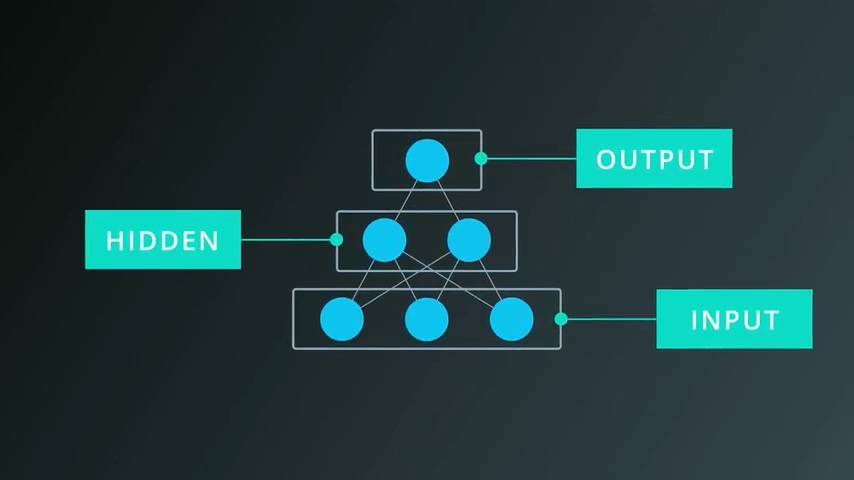1. 输入层
2. 输出层
3. 隐藏层（中间层）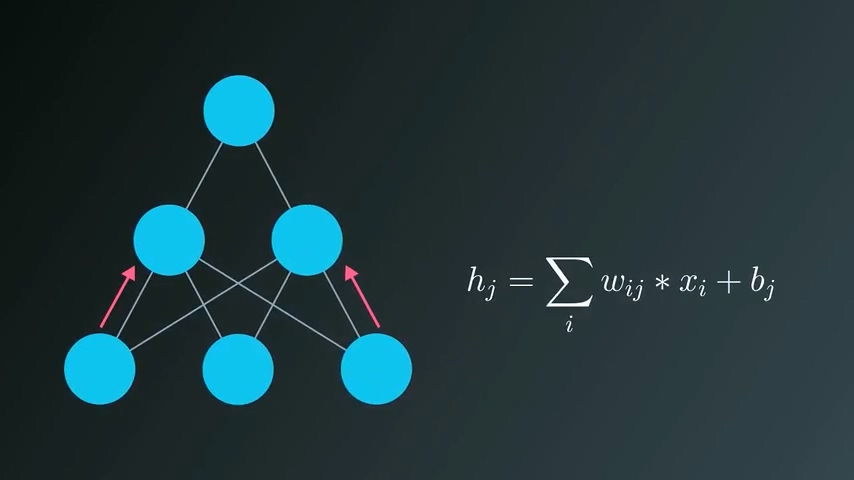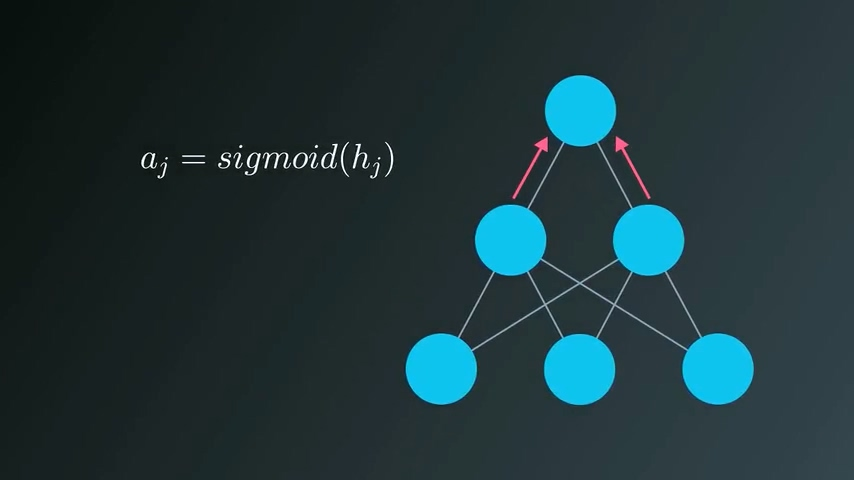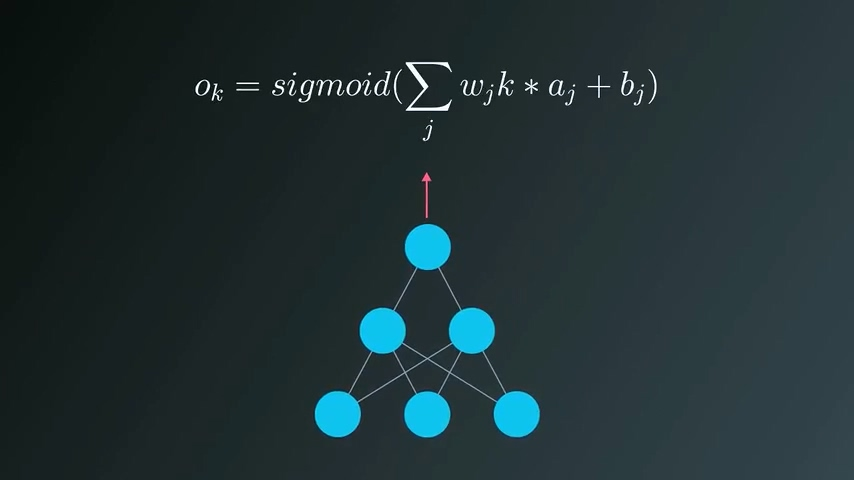# 集成隐藏层

## 起源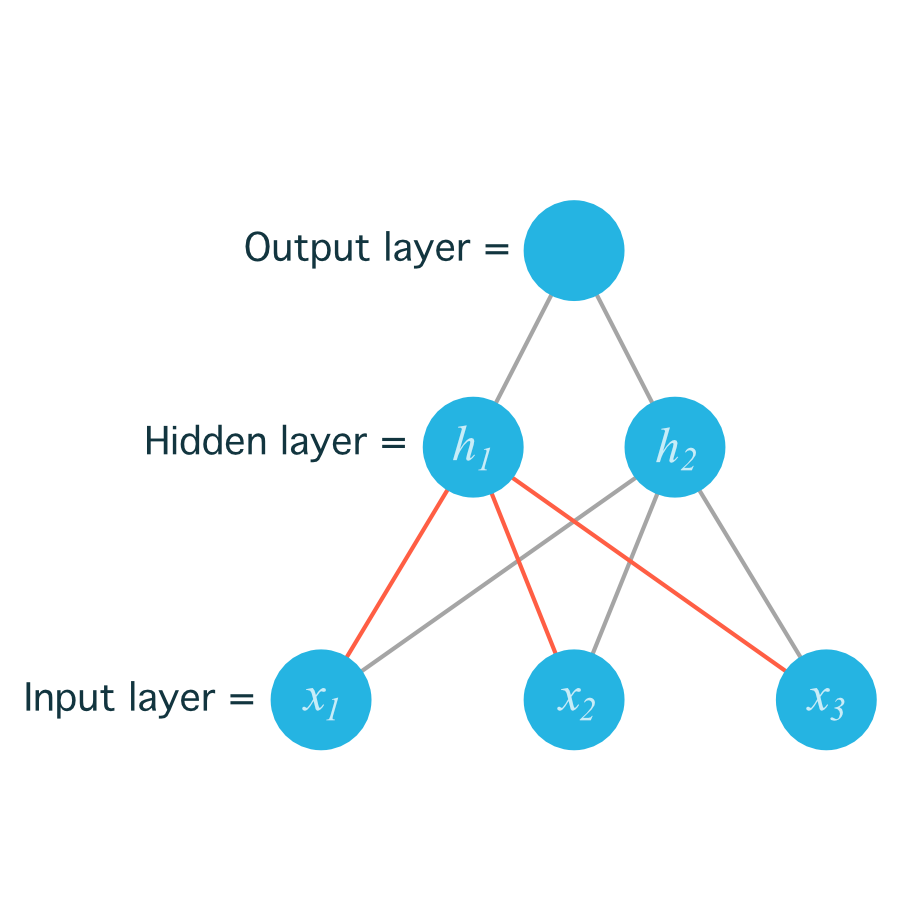– w11：从x1输入到h1的权重值
– w12：从x1输入到h2的权重值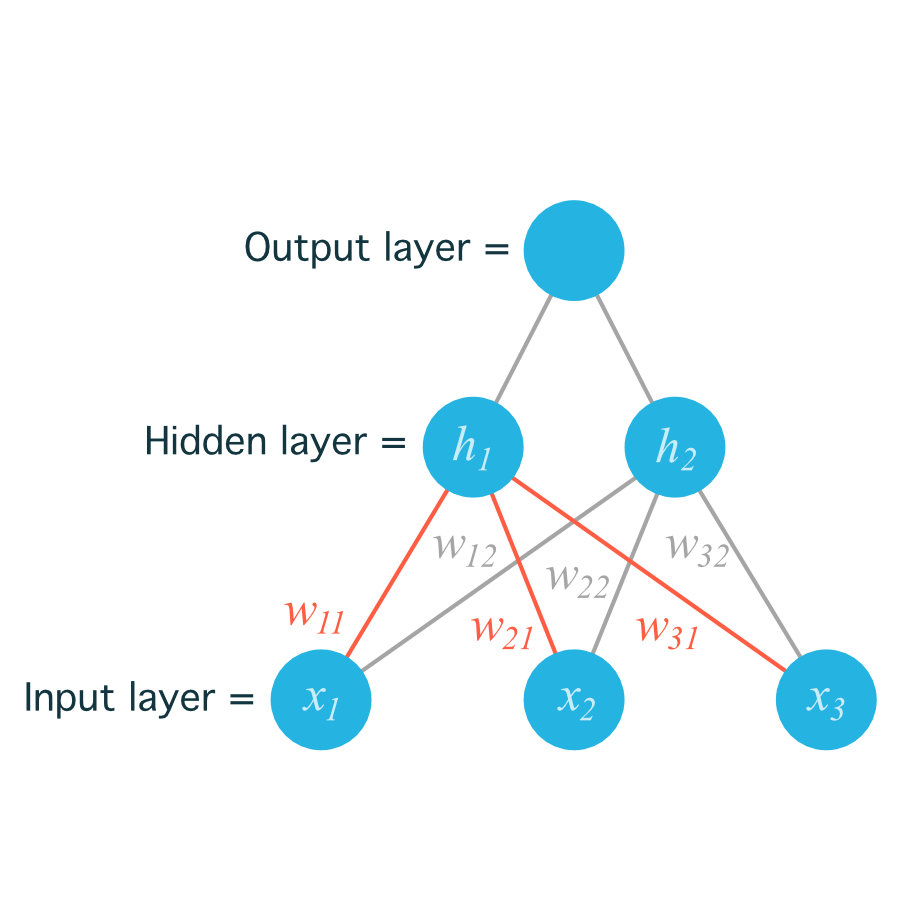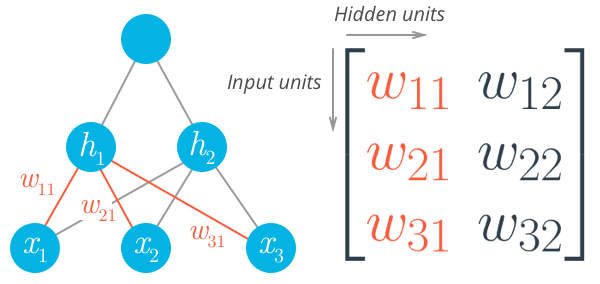``````# Number of records and input units
n_records, n_inputs = features.shape
# Number of hidden units
n_hidden = 2
weights_input_to_hidden = np.random.normal(0, n_inputs**-0.5, size=(n_inputs, n_hidden))
``````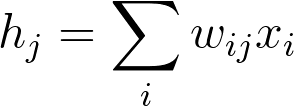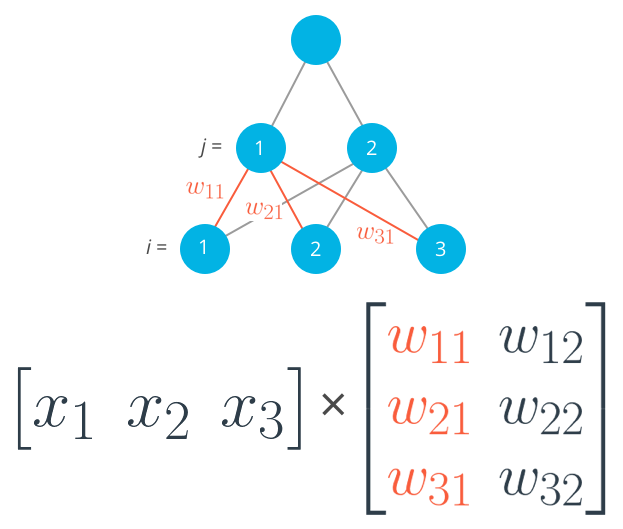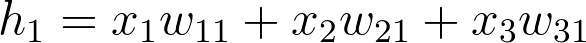``````hidden_inputs = np.dot(inputs, weights_input_to_hidden)
``````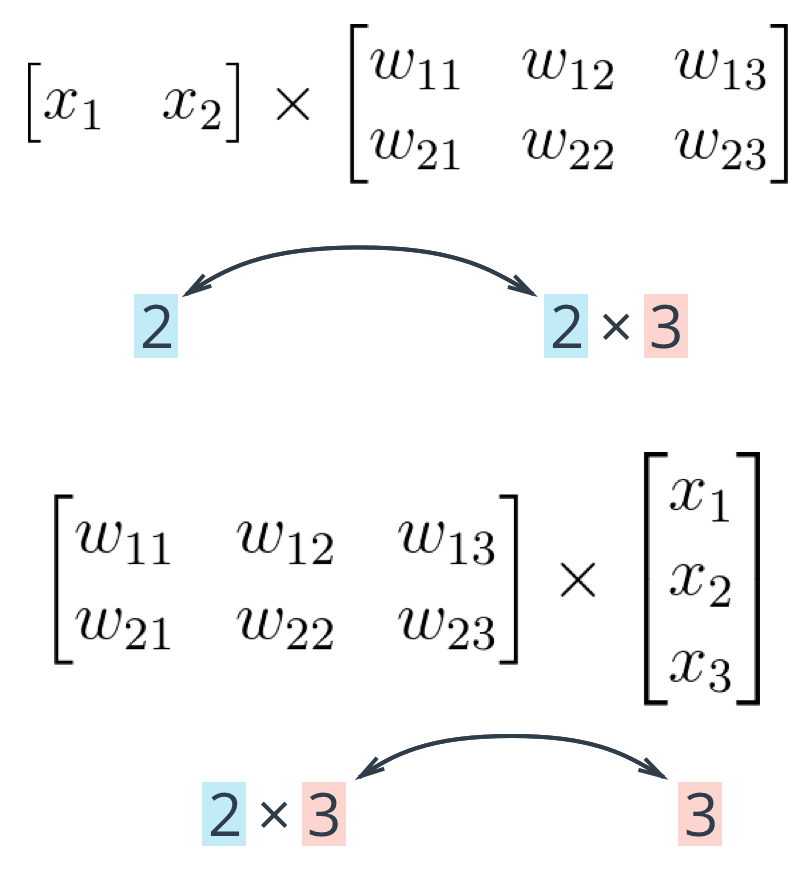# 生成列向量

``````print(features)
> array([ 0.49671415, -0.1382643 ,  0.64768854])

print(features.T)
> array([ 0.49671415, -0.1382643 ,  0.64768854])

print(features[:, None])
> array([[ 0.49671415],
[-0.1382643 ],
[ 0.64768854]])
``````

``````np.array(features, ndmin=2)
> array([[ 0.49671415, -0.1382643 ,  0.64768854]])

np.array(features, ndmin=2).T
> array([[ 0.49671415],
[-0.1382643 ],
[ 0.64768854]])
``````

# 编码

1. 计算输入层到隐藏层
2. 计算隐藏层的输出
3. 计算输出层的输入
4. 计算整个神经网络的输出

``````import numpy as np

def sigmoid(x):
"""
Calculate sigmoid
"""
return 1/(1+np.exp(-x))

# Network size
N_input = 4
N_hidden = 3
N_output = 2

np.random.seed(42)
# Make some fake data
X = np.random.randn(4)

weights_input_to_hidden = np.random.normal(0, scale=0.1, size=(N_input, N_hidden))
weights_hidden_to_output = np.random.normal(0, scale=0.1, size=(N_hidden, N_output))

# TODO: Make a forward pass through the network

hidden_layer_in = np.dot(X, weights_input_to_hidden)
hidden_layer_out = sigmoid(hidden_layer_in)

print('Hidden-layer Output:')
print(hidden_layer_out)

output_layer_in = np.dot(hidden_layer_out, weights_hidden_to_output)
output_layer_out = sigmoid(output_layer_in)

print('Output-layer Output:')
print(output_layer_out)
``````

0 回复

### 发表评论

Want to join the discussion?
Feel free to contribute!